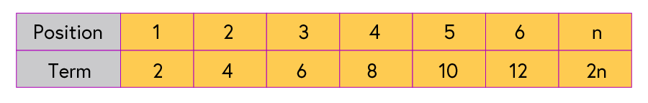## Want to keep learning?

This content is taken from the National STEM Learning Centre's online course, Maths Subject Knowledge: Understanding Numbers. Join the course to learn more.
4.3

# Sequences: term rules

Let’s clarify some of the terminology used to define sequences.

A sequence is usually denoted using a capital letter. The sequence $$E$$ is the sequence of even numbers 2,4,6,8, _, _, _, …

Each number in a sequence is called a term. The first term in this sequence is 2.

We write this as $$E_1 = 2$$ (the first term in the sequence E is equal to 2).

The nth term in the sequence is written as $$E_n$$.

We can explain how sequences are made using two different types of rules.

## Term to term rules

The term to term rule is a rule which tells you how to make the next term, if you already know one of the terms in a sequence. To generate the sequence we need two pieces of information.

We need one term of the sequence, this is usually the first term, and a rule which tells you how to get the next term.

For our sequence $$E$$ we would write:

$$E_1 = 2$$ (the first term is 2).
$$E_{n+1} = E_n + 2$$ (the next term equals the current term plus two).

## Position to term rules

A position to term rule is a formula which will generate the value of a term in a sequence determined by the position of the term within the sequence.

In our sequence of even numbers we have called $$E$$: 2,4,6,8,10,12,_, _, _ the first term is 2, the second term is 4 the third term is 6 and so on. We could write the sequence in a table.$$E_1 = 2$$, $$E_2 = 4$$, $$E_3 =6$$ and so on.

In the final box we write the position to term rule. In this case $$E_n = 2n$$

Often in school we are asked to find the nth term of a sequence. What we are really being asked to do is to find the position to term rule.

## The role of both rules

Consider the term to term rule and the position to term rule. Can you think of an advantage of the position to term rule over the term to term rule?

The position to term rule is useful if we just want to find the, say, 100th term of a sequence. The position to term rule allows us to do this (2 x 100 = 200) whereas using the term to term rule we need to find all the terms in the sequence up to the 100th term as we need the 99th term to find the 100th and the 98th to find the 99th and so on back to the first term.

The question we must ask is, if the position to term rule is better than the term to term rule, why do we bother with the term to term rule at all?

The term to term rule is very useful when studying iteration: a numerical method for solving equations. For some sequences, such as the Fibonacci sequence, the term to term is any easy way to describe the sequence whilst the position to term rule is complicated.